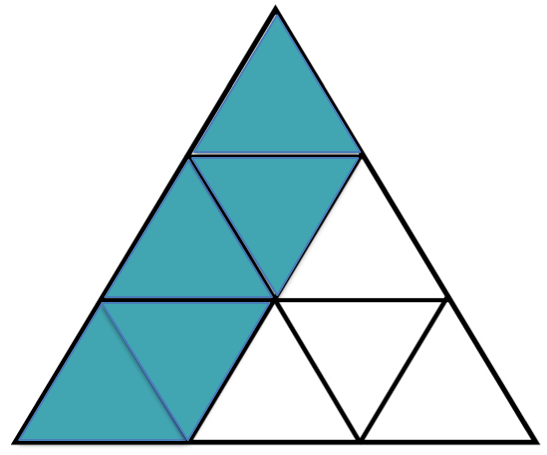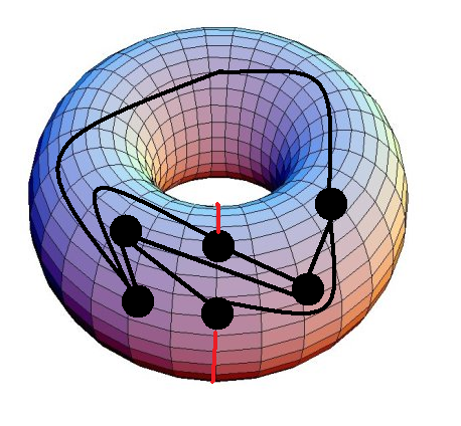Home About Us School Visits Elementary Schools Junior High Schools Senior High Schools Events at Dalhousie PD Day Workshops for Teachers (NEW) Contact Us
Junior High Schools

The below is a list of presentations appropriate for junior high school classes, in alphabetical order. If you would like us to come and give any of these presentations in your classroom for free, please use the contact form on the School Visits page.

Bothersome BrainteasersHas it been a real long time since you have 'troubled' yur brain in finding the right answer to a question? In this talk, you will experience four different brainteasers on shapes and patterns to tease your brain and have a good laugh too!

Outcomes:

• TBA.

This presentation is currently undergoing trials. If you are willing to host a trial-run in your classroom, please contact us.

Cryptography 1Cryptography is the study of protecting, coding, storing and transmitting information and messages so that only those who are intended to may read it. In other words, it is the study of secret messages and codes. Encryption is the conversion of messages to the secret code, called ciphertext. In order to read the information normally, on must decrypt the ciphertext, converting it back into plaintext. In this talk, we will look at some different types of cryptography that are used.

Outcomes:

• TBA.

This presentation is currently undergoing trials. If you are willing to host a trial-run in your classroom, please contact us.

Eulerian CircuitsIn this talk, students will be asked to find a route through the historic city of Königsberg so that they cross every bridge exactly once. This problem spawned an area of mathematics called graph theory, which makes this problem (and many others) much easier to solve! This talk serves as a gentle introduction to graph theory and its applications.

Outcomes:

• Solve problems using graphs.
• Learn definitions involving graphs.
• Look into different types of graphs.
• Try to deduce mathematical theorems.
• Understand why graph theory is so important in real life.

Fibonacci and the Golden RatioIn this talk students discover the relationship between the Golden Ratio and Fibonacci numbers. We also learn why the Golden Ratio is found throughout nature and listen to Fibonacci number inspired music.

Outcomes:

• What can happen when you sum numbers with a certain pattern.
• Look into a spatial math sequence and a special number, that appears in nature a lot and is now used in everyday life.
• Math and art.
• Math and music.
• Count the number of solutions to a problem.

Fractions FunWe introduce and explore the world of fractions! Using visual and real life examples as well as fraction based games we make fractions easy and fun.

Outcomes:

• Adding fractions with different denominators.
• Represent a given fraction concretely or pictorially.
• Using fraction addition, subtraction, multiplication, and division to solve problems.

This presentation is currently undergoing trials. If you are willing to host a trial-run in your classroom, please contact us.

Graph ColouringThis talk introduces students to graph theory. We look at the four colour problem, how graph colouring can be used to solve scheduling problems and even lead students through an activity that will classify what graphs can be coloured with 2 colours.

Outcomes:

• Solve problems using graphs.
• Look into different types of graphs.
• Try to deduce mathematical theorems.
• Understand why graph theory is so important in real life.
• Look into the known problem of map colouring.
• Applications to scheduling problems.

Jury DutyNEW: available also in French

A crime has been committed! By exploring various areas of mathematics, such as logic, graph theory, geometry and more we will see if we can put the guilty party behind bars!

Outcomes:

• Use math to solve problems that seem to have unpredictable solutions.
• Attempt to think outside the box to solve math problems.
• Determine and explain strategies for solving problems or winning games.
• Understand that a problem might not have a solution and being capable of explaining why.
• Apply logic to solve problems.
• Learn how to eliminate possible solutions to a problem.
• Introduction the mathematical areas that are currently studied: graph theory, cryptography, logic, geometry, algebra and number theory.

This presentation is currently undergoing trials. If you are willing to host a trial-run in your classroom, please contact us.

MathemagicNEW: available also in French

Can you always correctly guess someones birthday, magically untie yourself from ropes, or read someones mind? You should be able to! It is not magic, it's math!

Outcomes:

• Use math to solve problems that seem to have unpredictable solutions.
• Introduction to binary representation of numbers.
• Review of mental math skills and applying these to concrete problems.
• Factorization of large numbers.
• Use spatial skills to solve problems.

This presentation is currently undergoing trials. If you are willing to host a trial-run in your classroom, please contact us.

Nasty Number Tricks and Devious DivisibilityG.H. Hardy (a famous 20th Century mathematiian) wrote: 'Beauty is the first test: there is no permanent place in the world for ugly mathematics'.

Let us face it-algebra can be hard and there will be point for everyone when they find using algebra is difficult. But, believe it or not, algebra can be really beautiful and is, in fact, a powerful tool! Algebra can give a great sense of achievement every time a problem is solved and can, definitely, provide effective methods to break down and solve problems. In this talk, you will feel such a satisfaction and you will see how algebra allows you to take a situation and make it more general by examining nasty number tricks and devious divisibility.

Outcomes:

• TBA.

This presentation is currently undergoing trials. If you are willing to host a trial-run in your classroom, please contact us.

Prime NumbersLearn how ancient Greeks found prime numbers, and how huge primes today are the secret to internet encryption! We will learn some divisibility "tricks", prove that there are an infinite number of primes, and explore one of the oldest unsolved problems in mathematics: the Goldbach Conjecture!

Outcomes:

• Understand divisibility, factorization and prime numbers.
• Find solutions to problems by sieving.
• Why prime numbers are so important.

Problem SolvingNEW: available also in French

Explore the art of problem solving by investigating
tiling problems, number games and graph theory problems.

Outcomes:

• Use math to solve problems that seem to have unpredictable solutions.
• Attempt to think outside the box to solve math problems.
• Determine and explain strategies for solving problems or winning games.
• Understand that a problem might not have a solution and being capable of explaining why.
• Practice mental math skills.
• Recognize equivalent problems.
• View some interesting applications of areas and geometry.

TessellationsNEW: available also in French

We will explore geometry in artwork and nature through tessellations. We will look at the work of artist who used mathematics to enhance their work and even create some of our own tessellation artwork!

Outcomes:

• Counting possible solutions to a problem.
• Finding repeated patterns.Toads & Frogs is a game that is easy to play and fun to explore. We will find the number of moves needed to solve the game, and discover more about number patterns within the game.

Outcomes:

• Study the mathematics behind the strategies to a game.
• Use formulas to express number patterns.
• Introduce polynomials.

Tower of HanoiThe Tower of Hanoi is a very old and very popular problem. How many moves does it take to move a stack of disks from one peg to another, following certain rules? Legend has it that priests in a temple were solving the puzzle with 64 golden disks, and when the last move is completed, the world would end!

Outcomes:

• Study a classical game.
• Find number patterns within data.
• Use formulas to express number patterns.

Contact us for ideas on how we can best serve your needs!

Contact: Email our team at mathcircles@dal.ca

 Many Thanks to our Partners!Copyright 2015 www.nsmathcircles.com All Rights Reserved.